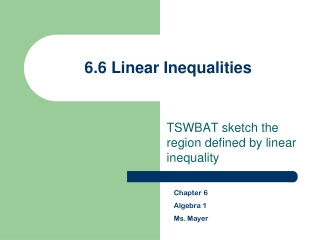DownloadDownload Presentation6.6 Linear Inequalities

# 6.6 Linear Inequalities

Télécharger la présentation## 6.6 Linear Inequalities

- - - - - - - - - - - - - - - - - - - - - - - - - - - E N D - - - - - - - - - - - - - - - - - - - - - - - - - - -
##### Presentation Transcript

1. 6.6 Linear Inequalities TSWBAT sketch the region defined by linear inequality Chapter 6 Algebra 1 Ms. Mayer

2. Overview • Graphing Linear Inequalities • solid line or dotted line • which side to shade • Writing Inequalities from a graph

3. Graphing Linear Inequalities in Two Variables How would you graph the equation x + y = 3? x + y = 3 -x -x y = -x + 3 m = -1 b = 3 What happens if the “=“ changes to “≤” or “≥”? We get shading!!!

4. Solid or dashed? The lines can be graphed as a solid line or a dashed (dotted) line. When do you think it’s solid, and when is it dashed? Think about graphing points on a number line; when is it open and when is it closed? < or > is … ≤ or ≥ is …

5. Graphing linear inequalities with two variables

6. Graphing Linear Inequalities Steps: 1. Graph the equation (solve for y) 2. Draw a Dotted or Solid line 3. Pick a point not on a line (any point) 4. Shade the correct side • True – shade the same side • False – shade the opposite side

7. Writing an Inequality Write an inequality for each graph. 5. 6.

8. Writing an Inequality Write an inequality for each graph. 7. 8.

9. Homework Graphing Inequalities • Solid line/Dotted line • Shading • HW: • 6.6 worksheet